# TIMEVALUE Function

Converts a text representation of a time to MS Excel time

## What is the TIMEVALUE Function?

The TIMEVALUE Function is categorized under Excel DATE/TIME functions. TIMEVALUE helps us convert a text representation of a time to MS Excel time.

In MS Excel, time is stored internally as a numerical value. It doesn’t recognize time in text form. Hence, the TIMEVALUE function allows us to convert the text representation of a time into a decimal that is recognizable as time.

In financial analysis, TIMEVALUE is useful when doing time calculations, such as hourly wages, or converting time imported from external sources in text form into a proper serial number of time.

### Formula

=TIMEVALUE(time_text)

The TIMEVALUE Function uses the following arguments:

Time_text (required argument) – This is a text string that represents a time. In the text string, we need to separate the hours, minutes and seconds by colons.

For example, if we provide just two values (separated by a colon) such as 04:54, TIMEVALUE will treat it as hours and minutes, not minutes and seconds. If we want to represent 4 minutes and 54 seconds, we need to specifically note hours as zero. That is as “00:04:54.”

### How to use the TIMEVALUE Function in Excel?

As a worksheet function, the TIMEVALUE Function can be entered as part of a formula in a cell of a worksheet.

To understand its uses, let’s consider a few examples:

#### Example 1

Let’s see the results we get in various text representations. The TIMEVALUE Function is used to convert text representations of times into decimal values that can be understood as times in Excel.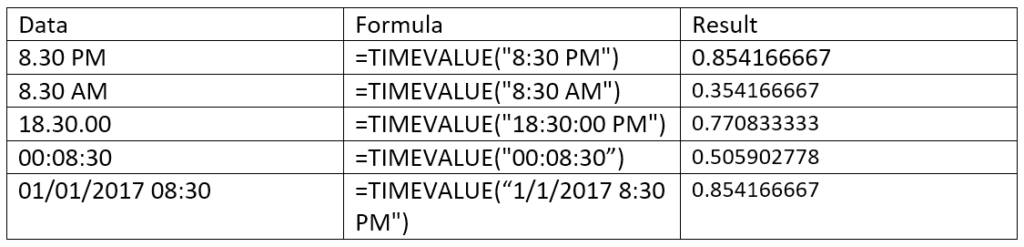The results we get in a spreadsheet are shown below:Cell A2 shows time in PM so the decimal returned for 8.30 pm, whereas in the next row, the decimal returned is for 8.30 am for the time in AM.

The value in cell A6 is a date and time. In this case, the TIMEVALUE function ignored the date part of the value and just used the time portion.

If we wish to calculate the difference between 8.30 PM and 8.30 AM, we can use the formula =TIMEVALUE(“8:30 PM”) – TIMEVALUE(“8:30 AM”). The result we would get is below: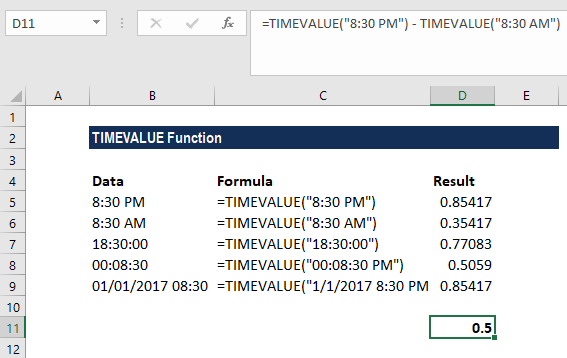As we know, times in Excel are regular decimal numbers formatted to look like times. And as they are numbers, we can add and subtract times just as with any other numerical values.

### Example 2

Suppose we imported data in the following format: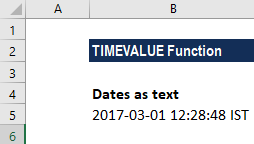Now we wish to put date and time in separate columns. For this, we can use the formulas =DATEVALUE(LEFT(B5,10)) and =TIMEVALUE(MID(B5,12,8)).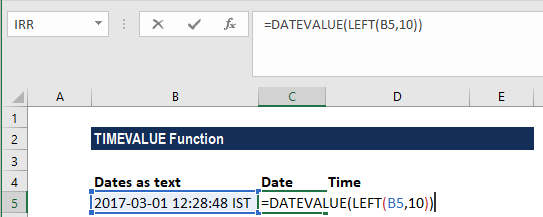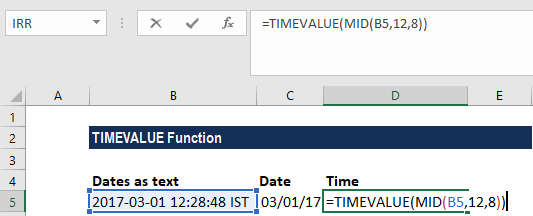To get the date, we extract the first 10 characters of the value with LEFT: so DATEVALUE(LEFT(B5,10)) will return the date as 03/01/17.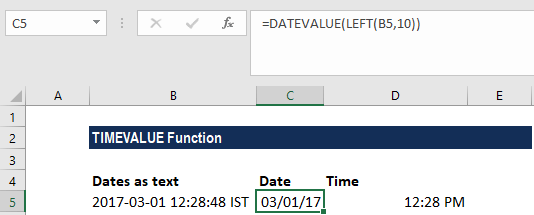The result is a text string. For Excel to interpret it as a date, we wrap LEFT in DATEVALUE, which converts the text into a proper Excel date value.

For the time, we extract 8 characters from the middle of the value with MID: MID(B5,12,8), which returns “12:28:48.” The result is in text form, so we use TIMEVALUE to interpret and convert it into Excel time.

### Things to remember about the TIMEVALUE function

1. TIMEVALUE was introduced in MS Excel 2000 and is available in all later versions.
2. #VALUE! error – Occurs when any of the given arguments cannot be recognized as a valid Excel time.

Thanks for reading CFI’s guide to important Excel functions! By taking the time to learn and master these functions, you’ll significantly speed up your financial analysis. To learn more, check out these additional CFI resources:

• Excel Functions for Finance